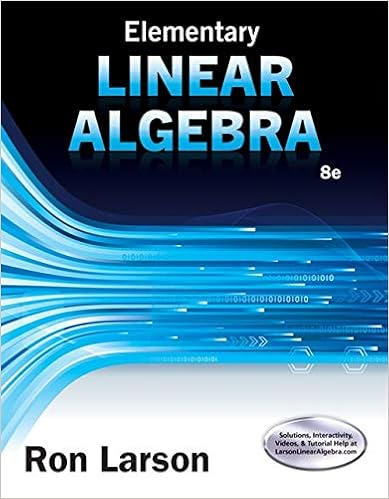# Eba alt tag angle eba 68 a 75 b 68 c 136 d 100

• Test Prep
• 46
• 99% (112) 111 out of 112 people found this document helpful

This preview shows page 27 - 30 out of 46 pages.

##### We have textbook solutions for you!
The document you are viewing contains questions related to this textbook.The document you are viewing contains questions related to this textbook.
Chapter 1 / Exercise 15
Elementary Linear Algebra
LarsonExpert Verified
EBA <alt tag = angle EBA> = 68° a. 75° *b. 68° c. 136° d. 100° Objective 8: Students will be able to apply properties of tangent-chord, chord-chord, and tangent-secant angles to solve problems. Display 2 Type: E 57) If m » AB <alt tag = arc AB> = 110°, calculate the m ABC <alt tag = angle ABC> . <alt tag = circle O with chord AB and tangent BC; the measure of angle ABC is x> a. An angle formed by an intersecting tangent and chord has its vertex on a point on the circle. The tangent-chord angle equals half the intersected arc. Since m » AB <alt tag = arc AB> = 270°, m ABC = <alt tag = angle ABC> 55°. Type: E 58) Calculate the m CLA
##### We have textbook solutions for you!
The document you are viewing contains questions related to this textbook.The document you are viewing contains questions related to this textbook.
Chapter 1 / Exercise 15
Elementary Linear Algebra
LarsonExpert Verified
a. LC and LA <alt tag = line segment LC and LA> are both tangents. The angle formed ( CLA <alt tag = angle CLA> ) = half the difference of the intercepted arcs ( ¼ AKC <alt tag = arc AKC> and » AC <alt tag = arc AC> ) m CLA <alt tag = angle CLA> = 1 2 <alt tag = one-half> (245° – 115°) m CLA <alt tag = angle CLA> = 65° Type: E 59) Calculate the m EBA <alt tag = angle EBA> , given m » DA <alt tag = arc DA> )= 98° and m ¼ EFA <alt tag = arc EFA> = 262° <alt tag = circle C with secant BE and tangent BA; secant BE intersects circle C at point D; point F is on the edge of circle C between points A and E and opposite D> a. BE <alt tag = line segment BE> is a secant and BF <alt tag = line segment BF> is a tangent. The angle formed ( EBA <alt tag = angle EBA> ) = half the difference of the intercepted arcs ( ¼ EFA <alt tag = arc EFA> and » DA <alt tag = arc DA> ). m EBA <alt tag = angle EBA> = 1 2 <alt tag = one-half> (262° – 98°) m EBA <alt tag = angle EBA> = 82° Type: E 60) Calculate the m EBF <alt tag = angle EBF> , given m » DA <alt tag = arc DA> = 35° and m º EF <alt tag = arc EF> = 100°
<alt tag = circle C with secants BE and BF forming angle EBF; secant BE intersect circle C at point B and secant BF intersects circle C at point A> a. BE and BF <alt tag = line segment BE and BF> are both secants. The angle formed ( EBF <alt tag = angle EBF> ) = half the difference of the intercepted arcs ( º EF <alt tag = arc EF> and » DA <alt tag = arc DA> ) m EBF <alt tag = angle EBF> = 1 2 <alt tag = one-half> (100° – 35°) m EBF <alt tag = angle EBF> = 32.5° Objective 9: Students will be able to write the standard equation of a circle Display 1 61) What is the standard equation of a circle? @ (x – h) 2 + (y – k) 2 = r 2
•••